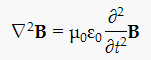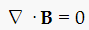# Q & A: Can electromagnetic waves have magnetic monopoles?

Q:
The wave equation for light is a simple sine function for both E and B, and the divergence of B isn't zero. Is this a magnetic monopole?
- Patrick (age 35)
Boston, MA, USA
A:

Hi Patrick,

The usual wave equation for the magnetic field of light isThe equation for the electric field is identical, but E is replaced by B. The operator on the left side is the Laplacian: the divergence of the gradient of B. This is NOT the divergence of B itself, which IS zero, an assumption used in deriving the above equation (and verified by all experiments to date).This equation, as you know, means that there are no magnetic monopoles, and as far as we know is true for all situations in our universe. So light waves, like all other electric or magnetic fields, obey this equation.

By the way, sine waves are solutions to the wave equation, but there are many different solutions also. None of these solutions have B fields with nonzero divergence.

David Schmid

(published on 05/13/2014)

## Follow-Up #1: no divergence of magnetic field

Q:
That means the magnetic field lines should on itself. But in an EM wave, how does this happen?.
- John (age 27)
Ernakulam, Kerala, India
A:

Nice question. The answer will depend on what sort of wave pattern is being described. Let's look at a simple example, a wave from an oscillating vertical electric dipole. In any direction in the horizontal plane at right angles to the oscillation, the wave propagates outward with a vertical E and a horizontal BB points along the circle centered at the dipole. The B fields in that plane just form closed circles.

Mike W.

(published on 12/12/2016)